# [4.MD.4-1.0] Fractional Line Plots - Common Core Standard

##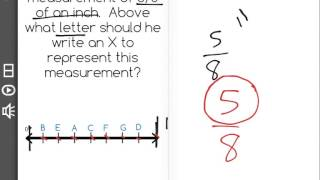By Freckle education

Make a line plot to display a data set of measurements in fractions of a unit (1/2, 1/4, 1/8)# Line plots and stem-and-leaf plots

##By MathPlanetVideos

Construct a stem-and-leaf plot out of the following data: 6, 6,7,8,9,13,16,19,21,25,26# Line Plots with Fractional Data

##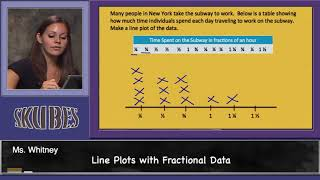By Skubes ed

Make a line plot to display a data set of measurements in fractions of a unit (1/2, 1/4, 1/8)# Understanding Measurement Data Displayed in Line Plots: 2.MD.9

##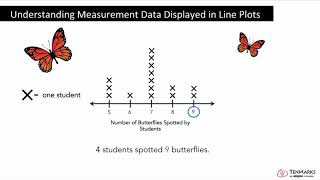By Tenmarks Amazon

Students learn how to interpret measurement data displayed in line plots.# Fractions and Line Plots

##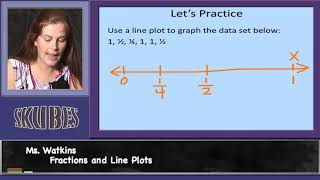By Skubes ed

Use the four operations to solve word problems involving distances, intervals of time, liquid volumes, masses of objects, and money, including problems involving simple fractions or decimals, and problems that require expressing measurements given in a larger unit in terms of a smaller unit.# Line Plots for Fractions

##By Icon Math

Make line plots to display measurements in fractions of a unit.# Interpreting Line plots

##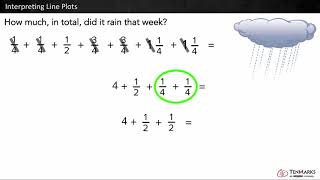By Tenmarks Amazon

Students learn to interpret line plots by using the given data to answer questions involving fractions and whole numbers.# Introduction to line plots | Measurement and data | Early Math | Khan Academy

##By KhanAcademy##By Anywhere Math